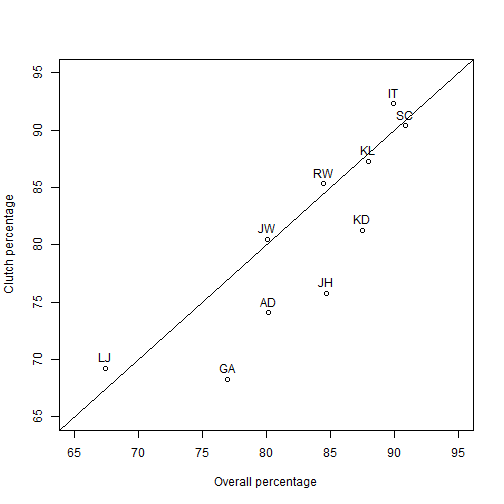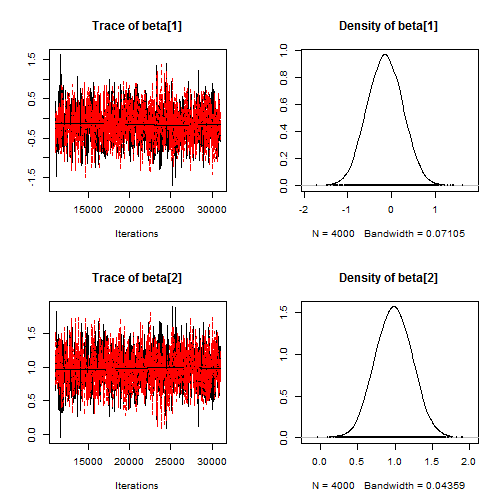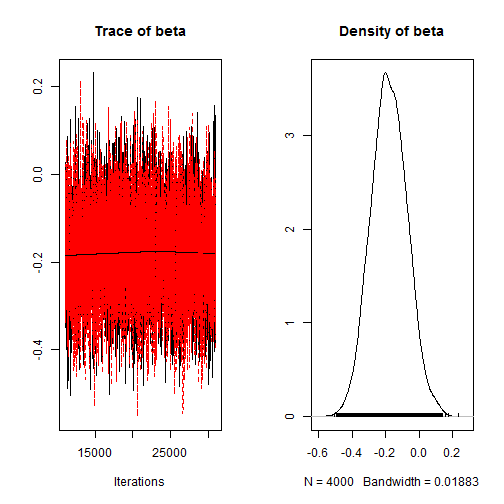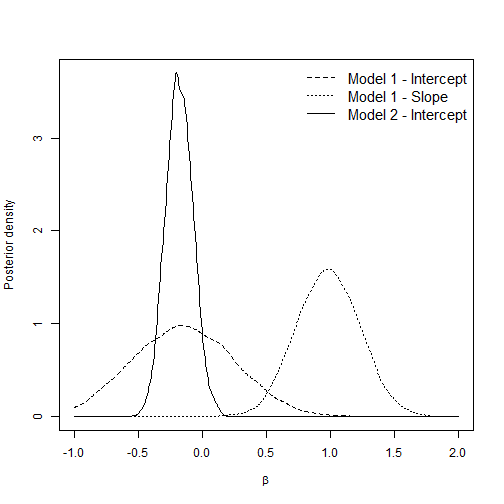# Logistic regression for NBA clutch free throws

### Chapter 4.3.3: Generalized linear models

The NBA clutch free throws data set has three variables for player $$i=1,…,10$$:

1. $$Y_i$$ is the number clutch free throws made
2. $$N_i$$ is the number clutch free throws attempted
3. $$q_i$$ is the proportion of the non-clutch free throws made

We model these data as $Y_i\sim\mbox{Binomial}(N_i,p_i),$ where $$p_i$$ is the true probability of making a clutch shot. The objective is to explore the relationship between clutch and overall percentages, $$p_i$$ and $$q_i$$. We do this using two logistic regression models:

1. $$\mbox{logit}(p_i) = \beta_1 + \beta_2\mbox{logit}(q_i)$$
2. $$\mbox{logit}(p_i) = \beta_1 + \mbox{logit}(q_i)$$

In both models we select uninformative priors $$\beta_j\sim\mbox{Normal}(0,10^2)$$.

In the first model, $$p_i=q_i$$ if $$\beta_1=0$$ and $$\beta_2=1$$; in the second model $$p_i=q_i$$ if $$\beta_1=0$$. Therefore, we compare the posteriors of the $$\beta_j$$ to these values to analyze the relationship between $$p_i$$ and $$q_i$$.

set.seed(0820)

Y <- c(64, 72, 55, 27, 75, 24, 28, 66, 40, 13)
N <- c(75, 95, 63, 39, 83, 26, 41, 82, 54, 16)
q <- c(0.845, 0.847, 0.880, 0.674, 0.909, 0.899, 0.770, 0.801, 0.802, 0.875)

X <- log(q)-log(1-q) # X = logit(q)


## Plot the data

inits   <- c("RW","JH","KL","LJ","SC","IT","GA","JW","AD","KD")
plot(100*q,100*Y/N,
xlim=100*c(0.65,0.95),ylim=100*c(0.65,0.95),
xlab="Overall percentage",ylab="Clutch percentage")
text(100*q,100*Y/N+1,inits)
abline(0,1)## Fit the first model in JAGS

 library(rjags)

## Loading required package: coda

## Linked to JAGS 4.2.0

## Loaded modules: basemod,bugs

 data   <- list(Y=Y,N=N,X=X)
params <- c("beta")

model_string <- textConnection("model{
# Likelihood
for(i in 1:10){
Y[i]        ~ dbinom(p[i],N[i])
logit(p[i]) <- beta + beta*X[i]
}
# Priors
beta ~ dnorm(0,0.01)
beta ~ dnorm(0,0.01)
}")

model <- jags.model(model_string,data = data, n.chains=2,quiet=TRUE)
update(model, 10000, progress.bar="none")
samples1 <- coda.samples(model, variable.names=params, thin=5, n.iter=20000, progress.bar="none")

plot(samples1)summary(samples1)

##
## Iterations = 11005:31000
## Thinning interval = 5
## Number of chains = 2
## Sample size per chain = 4000
##
## 1. Empirical mean and standard deviation for each variable,
##    plus standard error of the mean:
##
##            Mean     SD Naive SE Time-series SE
## beta -0.1486 0.4045 0.004522        0.01294
## beta  0.9826 0.2481 0.002774        0.00787
##
## 2. Quantiles for each variable:
##
##            2.5%     25%     50%    75%  97.5%
## beta -0.9239 -0.4265 -0.1490 0.1275 0.6359
## beta  0.5071  0.8132  0.9827 1.1517 1.4694

 b1 <- c(samples1[][,1],samples1[][,1])
b2 <- c(samples1[][,2],samples1[][,2])


## Fit the second model in JAGS

 model_string <- textConnection("model{
# Likelihood
for(i in 1:10){
Y[i]        ~ dbinom(p[i],N[i])
logit(p[i]) <- beta + X[i]
}
# Priors
beta ~ dnorm(0,0.01)
}")

model <- jags.model(model_string,data = data, n.chains=2,quiet=TRUE)
update(model, 10000, progress.bar="none")
samples2 <- coda.samples(model, variable.names=params, thin=5, n.iter=20000, progress.bar="none")
b3 <- c(samples2[],samples2[])

plot(samples2)summary(samples2)

##
## Iterations = 11005:31000
## Thinning interval = 5
## Number of chains = 2
## Sample size per chain = 4000
##
## 1. Empirical mean and standard deviation for each variable,
##    plus standard error of the mean:
##
##           Mean             SD       Naive SE Time-series SE
##      -0.179428       0.107197       0.001199       0.001198
##
## 2. Quantiles for each variable:
##
##     2.5%      25%      50%      75%    97.5%
## -0.38598 -0.25251 -0.18186 -0.10721  0.03334

 mean(b3<0) # Prob(beta_3<0|Y)

##  0.95275


## Plot the posterior densities from both models

 d1 <- density(b1,from=-1,to=2)
d2 <- density(b2,from=-1,to=2)
d3 <- density(b3,from=-1,to=2)

mx <- max(c(d1$y,d2$y,d3$y)) plot(d3$x,d3$y,type="l",xlim=c(-1,2),ylim=c(0,mx),xlab=expression(beta),ylab="Posterior density") lines(d1$x,d1$y,lty=2) lines(d2$x,d2\$y,lty=3)

legend("topright",c("Model 1 - Intercept","Model 1 - Slope","Model 2 - Intercept"),
bty="n",lty=c(2,3,1),cex=1.25)Summary: In the second model, we find that $$\beta_1$$ is negative with posterior probability around 0.95. If $$\beta_1$$ is negative this implies that the clutch probability is less than the overall probability. Therefore, there is some evidence that free throw percentage decreases in clutch situations.